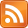SMS scnews item created by Daniel Daners at Fri 23 Mar 2018 1614
Type: Seminar
Distribution: World
Expiry: 27 Mar 2018
Calendar1: 27 Mar 2018
CalLoc1: AGR Carslaw 829
CalTitle1: PDE Seminar: Sharp bounds for Neumann eigenvalues (Brandolini)
Auth: daners@dora.maths.usyd.edu.au

# Sharp bounds for Neumann eigenvalues

### Brandolini

Barbara Brandolini
Università degli Studi di Napoli “Federico II”, Italy
Tue 27th Mar 2018, 2-3pm, Carslaw Room 829 (AGR)

## Abstract

We prove a sharp lower bound for the first nontrivial Neumann eigenvalue ${\mu }_{1}\left(\Omega \right)$ of the $p$-Laplace operator ($p>1$) in a Lipschitz, bounded domain $\Omega$ in ${ℝ}^{n}$. Differently from the pioneering estimate by Payne-Weinberger, our lower bound does not require any convexity assumption on $\Omega$, it involves the best isoperimetric constant relative to $\Omega$ and it is sharp, at least when $p=n=2$, as the isoperimetric constant relative to $\Omega$ goes to 0. Moreover, in a suitable class of convex planar domains, our estimate turns out to be better than the one provided by the Payne-Weinberger inequality.

Furthermore, we prove that, when $p=n=2$ and $\Omega$ consists of the points on one side of a smooth curve $\gamma$, within a suitable distance $\delta$ from it, then ${\mu }_{1}\left(\Omega \right)$ can be sharply estimated from below in terms of the length of $\gamma$, the ${L}^{\infty }$ norm of its curvature and $\delta$.

For Seminar announcements you can now subscribe to theSeminar RSS feed. Check also the PDE Seminar page.

Enquiries to Daniel Hauer or Daniel Daners.